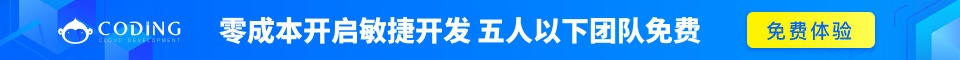# Django Ajax

```<!DOCTYPE html>
<html>
<body>
<p>请输入两个数字</p>
a: <input type="text" id="a" name="a"> <br>
b: <input type="text" id="b" name="b"> <br>
<p>result: <span id='result'></span></p>
<button type="button" id='sum'>提交</button>
</form>

<script src="http://apps.bdimg.com/libs/jquery/1.11.1/jquery.min.js"></script>
<script>
\$("#sum").click(function(){
var a = \$("#a").val();
var b = \$("#b").val();

\$('#result').html(ret)
})
});
});
</script>
</body>
</html>```views.py 中可以用  request.is_ajax() 方法判断是否是 ajax 请求，需要添加一个 HTTP 请求头：

`xmlhttp.setRequestHeader("X-Requested-With", "XMLHttpRequest");`

`用 \$.ajax 方法代替 \$.get，因为 \$.get 在 IE 中不会发送 ajax header`

```STANDARD_HEADERS = ['REFER', 'HOST', ...] # just for example

value = value.replace('-', '_').upper()

return value

return 'HTTP_' + value```

views.py

```from django.http import HttpResponse
import json

def ajax_list(request):
a = range(100)
return HttpResponse(json.dumps(a), content_type='application/json')

def ajax_dict(request):
name_dict = {'twz': 'Love python and Django', 'zqxt': 'I am teaching Django'}
return HttpResponse(json.dumps(name_dict), content_type='application/json')```

Django 1.7 及以后的版本有更简单的方法（使用 JsonResponse(官方文档))：

```from django.http import JsonResponse

def ajax_list(request):
a = range(100)
return JsonResponse(a, safe=False)

def ajax_dict(request):
name_dict = {'twz': 'Love python and Django', 'zqxt': 'I am teaching Django'}
return JsonResponse(name_dict)```

```from django.http import HttpResponse

import json

class JsonResponse(HttpResponse):
def __init__(self,
content={},
mimetype=None,
status=None,
content_type='application/json'):

super(JsonResponse, self).__init__(
json.dumps(content),
mimetype=mimetype,
status=status,
content_type=content_type)```

```    url(r'^ajax_list/\$', 'tools.views.ajax_list', name='ajax-list'),
url(r'^ajax_dict/\$', 'tools.views.ajax_dict', name='ajax-dict'),``````<!DOCTYPE html>
<html>
<body>
<p>请输入两个数字</p>
a: <input type="text" id="a" name="a"> <br>
b: <input type="text" id="b" name="b"> <br>
<p>result: <span id='result'></span></p>
<button type="button" id='sum'>提交</button>
</form>

<div id="dict">Ajax 加载字典</div>
<p id="dict_result"></p>

<div id="list">Ajax 加载列表</div>
<p id="list_result"></p>

<script src="http://apps.bdimg.com/libs/jquery/1.11.1/jquery.min.js"></script>
<script>
// 求和 a + b
\$("#sum").click(function(){
var a = \$("#a").val();
var b = \$("#b").val();

\$('#result').html(ret);
})
});

// 列表 list
\$('#list').click(function(){
\$.getJSON('/ajax_list/',function(ret){
//返回值 ret 在这里是一个列表
for (var i = ret.length - 1; i >= 0; i--) {
// 把 ret 的每一项显示在网页上
\$('#list_result').append(' ' + ret[i])
};
})
})

// 字典 dict
\$('#dict').click(function(){
\$.getJSON('/ajax_dict/',function(ret){
//返回值 ret 在这里是一个字典
\$('#dict_result').append(ret.twz + '<br>');
// 也可以用 ret['twz']
})
})
});
</script>
</body>
</html>```

```<script>
// 求和 a + b
\$("#sum").click(function(){
var a = \$("#a").val();
var b = \$("#b").val();

\$('#result').html(ret);
})
});

// 列表 list
\$('#list').click(function(){
\$.getJSON("{% url 'ajax-list' %}",function(ret){
//返回值 ret 在这里是一个列表
for (var i = ret.length - 1; i >= 0; i--) {
// 把 ret 的每一项显示在网页上
\$('#list_result').append(' ' + ret[i])
};
})
})

// 字典 dict
\$('#dict').click(function(){
\$.getJSON("{% url 'ajax-dict' %}",function(ret){
//返回值 ret 在这里是一个字典
\$('#dict_result').append(ret.twz + '<br>');
// 也可以用 ret['twz']
})
})
});
</script>```

```person_info_dict = [
{"name":"xiaoming", "age":20},
{"name":"tuweizhong", "age":24},
{"name":"xiaoli", "age":33},
]```

\$.each() 方法代替 for 循环，html 代码（jQuery)

```\$.getJSON('ajax-url-to-json', function(ret) {
\$.each(ret, function(i,item){
// i 为索引，item为遍历值
});
});```

```\$.getJSON('ajax-get-a-dict', function(ret) {
\$.each(ret, function(key, value){
// key 为字典的 key，value 为对应的值
});
});```↑ 上方为自强学堂赞助商，非常荣幸有他们支持自强学堂的发展，感兴趣的了解一下他们的产品。